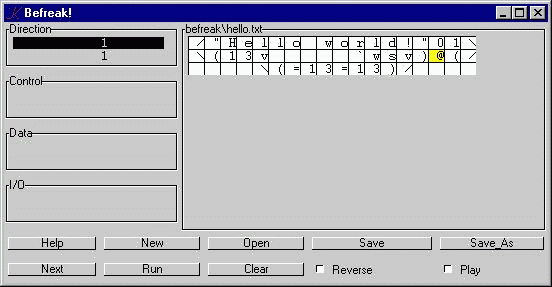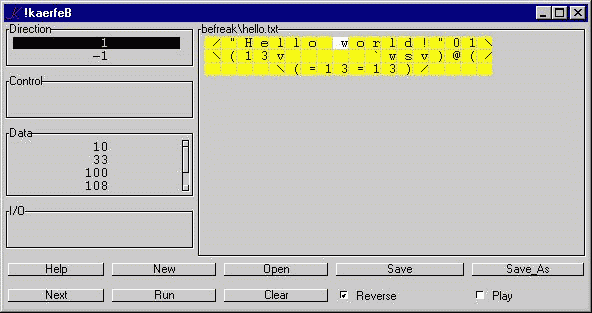# Befreak in K

## Befreak

Befreak is a two-dimensional reversible programming language invented by Brent Kerby and Hilton Campbell.

Go here for their original documentation and implementation, and here for the K implementation.

## Running the K version

Kick it off by saying

`	k befreak`

or

`	k befreak program.txt`

Click 'Help' for help:In the K version, I use 'm' for greater-than. 'l' and 'm' make a nice pair ("less" and "more"), and this choice frees up 'g' for get (to get a token from the current program rectangle) and 'p' for put (to put a token into the current program rectangle). Not yet implemented.

Features will be added on an irregular basis. Or maybe not.

Assuredly, there are bugs, but "Hello World!" and primes generation work as advertised.

## Implementation

### Globals

```B		the numerics buffer, a character vector.
C		control stack, an integer vector.
D		data stack, an integer vector.
E 		input/output stack, an integer vector.
I		instruction pointer: P . I is the current instruction.
J  		J is 0 (rows) if the direction is north/south, 1 (cols) if west/east.
J is 1 (south/east) or -1 (north/west).
K 		list of four two-element vectors: (north;south;west;east).
P		the current program, a character matrix.
R		reverse bit, 0 if normal mode, 1 if reverse mode.
S 		string-mode bit, 0 if normal mode, 1 if accumulating the characters of a string.
T 		timer bit.
V		stop list.
W 		the GUI, a dictionary.
X 		matrix of the Befreak instructions.
Y		the current file, a character vector.
Z		run flag: 0 (not running) or 1 (running).```

### 'step' and its subfunctions

The Befreak evaluator is called "step":

`step:{:["\""=t:P . I;q[];S;s t;a t];if[Z;i[]]}`

The function is a single case-statement with a continuation condition at the end:

`i:{I[*J]+:J 1;I::I!'^P}`

The instruction pointer "wraps" at the edges of the program rectangle.

If the current instruction-token P . I matches the quote-mark, we call q, which toggles the string-mode flag. Within q, we test to see if we've just entered string mode, and if so, we call the numerics buffer-handler function n:

`q:{if[S~:;n[]]}`

Else, if we're in string mode (S is non-zero), we call s, which appends the ascii value of the character to the data stack:

`s:{D,:_ic x}`

Else we call the instruction-processor a:

`a:{if[~x _in"0123456789";n[]];X[X[;0;R]?x;1]x}`

If x is not numeric, we call the numerics buffer-handler. Then we find and apply the instruction for x to x. In most cases, the instruction will ignore the argument-value, but in the case of a numeric constant, e.g. "7", the instruction will push "7" onto the numerics buffer.

The numerics buffer-handler is:

`n:{if[#B;if[~#D;D,:0];D[-1+#D]:xor[*|D;0\$B];B::""]`

If the buffer is non-empty, then we xor its numeric value with the top of the data-stack. (If the data-stack is empty, we silently append a 0.)

The Befreak instructions are contained in the matrix X. X[;0] is a two column matrix of instruction characters. E.g. "%*" is one such. If reverse mode is 0, then we execute the function corresponding to X[;0;0]?t, else we execute the function corresponding to X[;0;1]?t, where t is the current instruction token.

## GUI

`k befreak``k befreak hello.txt`Press 'Next' a few times:Press 'Reverse':